Introduction Intended Audience This St AIR is intended

• Slides: 65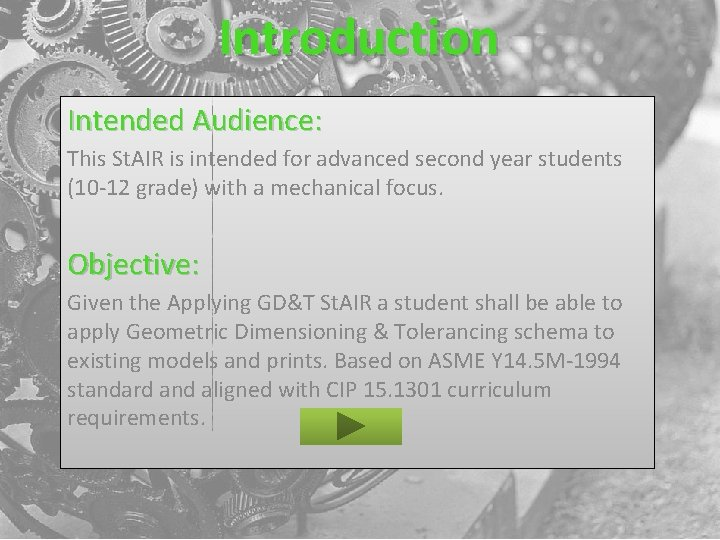Introduction Intended Audience: This St. AIR is intended for advanced second year students (10 -12 grade) with a mechanical focus. Objective: Given the Applying GD&T St. AIR a student shall be able to apply Geometric Dimensioning & Tolerancing schema to existing models and prints. Based on ASME Y 14. 5 M-1994 standard and aligned with CIP 15. 1301 curriculum requirements.Applying GD&T Purpose: Today you will be reviewing both the Linear tolerancing scheme you have been exposed to as well as being introduced to a new form of tolerancing and begin applying it on our prints. That form of tolerancing is called Geometric Dimensioning & Tolerancing or Geometric Dimensioning & Tolerancing simply GD&T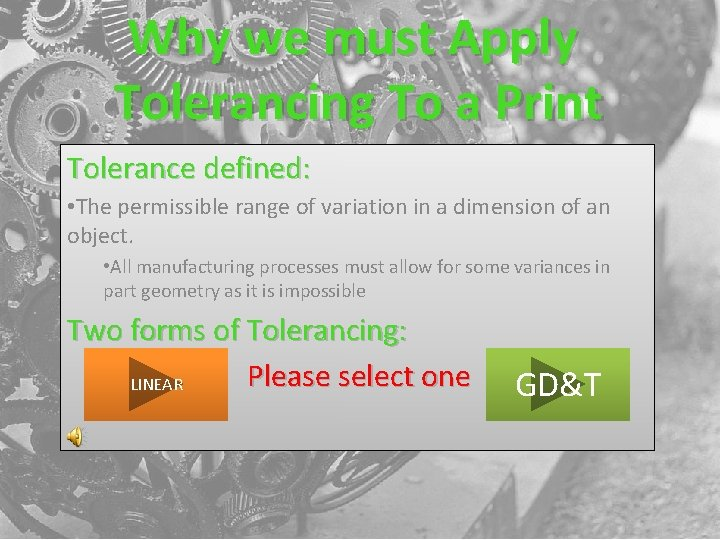Why we must Apply Tolerancing To a Print Tolerance defined: • The permissible range of variation in a dimension of an object. • All manufacturing processes must allow for some variances in part geometry as it is impossible Two forms of Tolerancing: Please select one LINEAR GD&TLinear Tolerancing • Based on X&Y coordinates creating a rectangular tolerance zone for part position. • Example of tolerance zone • TYPES OF LINEAR TOLERANCES: BILATERAL UNILATERAL PLUS AND MINUS LIMIT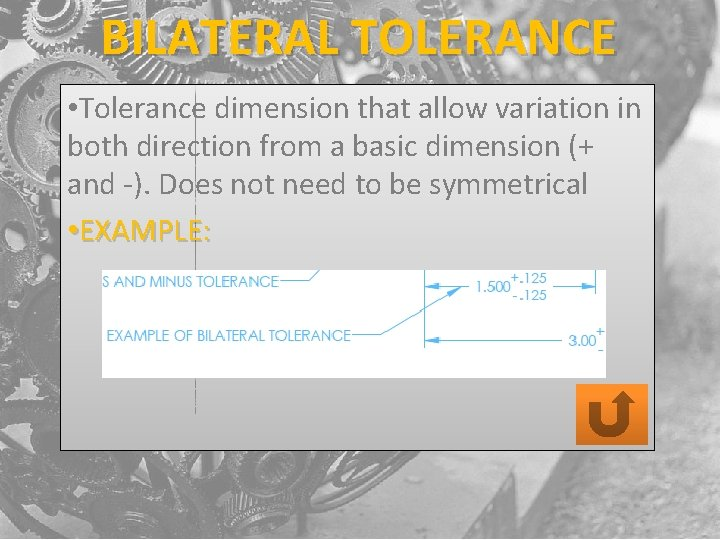BILATERAL TOLERANCE • Tolerance dimension that allow variation in both direction from a basic dimension (+ and -). Does not need to be symmetrical • EXAMPLE:UNILATERAL TOLERANCE • Tolerance dimension that allow variation in only one direction from a basic dimension (+ or -). • EXAMPLES:PLUS/MINUS TOLERANCE • Tolerance dimension that used to indicate the tolerance range above and below the basic dimension. Must remain symmetrical • EXAMPLE: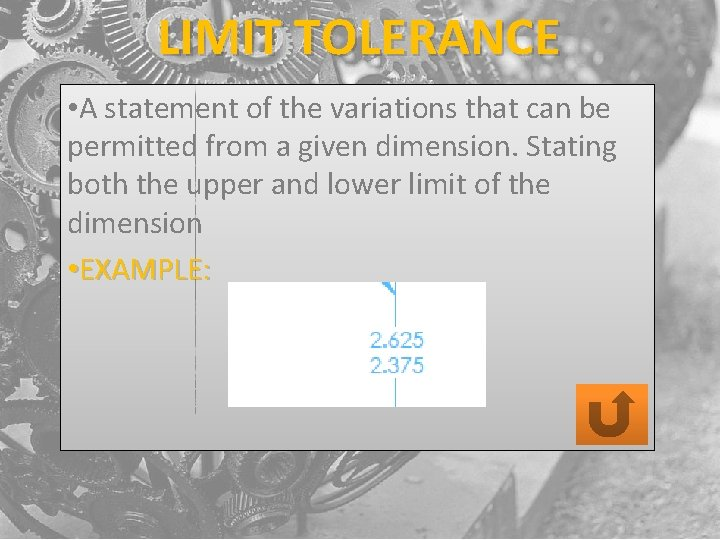LIMIT TOLERANCE • A statement of the variations that can be permitted from a given dimension. Stating both the upper and lower limit of the dimension • EXAMPLE:LINEAR TOLERANCE ZONE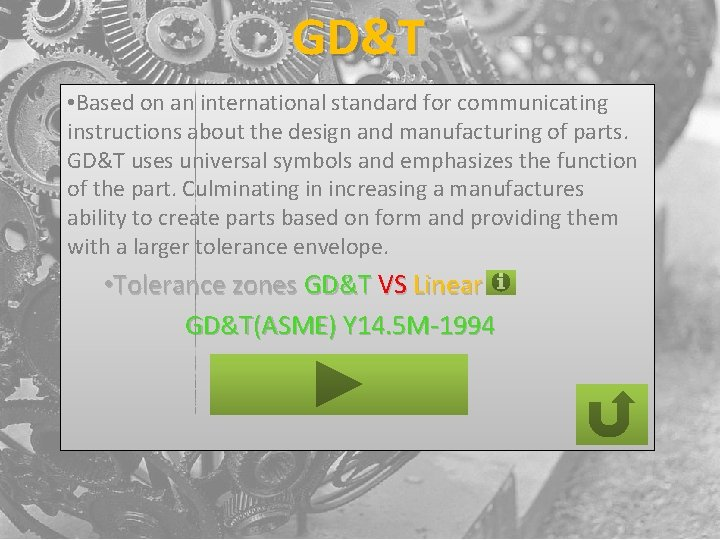GD&T • Based on an international standard for communicating instructions about the design and manufacturing of parts. GD&T uses universal symbols and emphasizes the function of the part. Culminating in increasing a manufactures ability to create parts based on form and providing them with a larger tolerance envelope. • Tolerance zones GD&T VS Linear GD&T(ASME) Y 14. 5 M-1994TOLERANCE ZONES GD&T VS LINEAR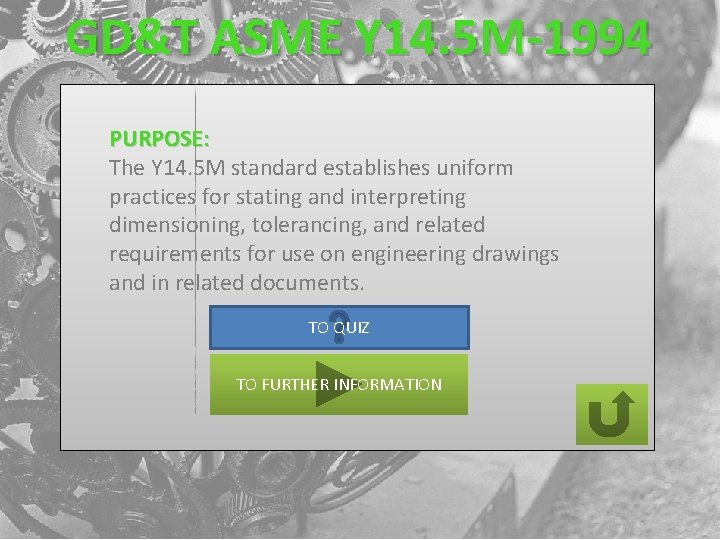GD&T ASME Y 14. 5 M-1994 PURPOSE: The Y 14. 5 M standard establishes uniform practices for stating and interpreting dimensioning, tolerancing, and related requirements for use on engineering drawings and in related documents. TO QUIZ TO FURTHER INFORMATION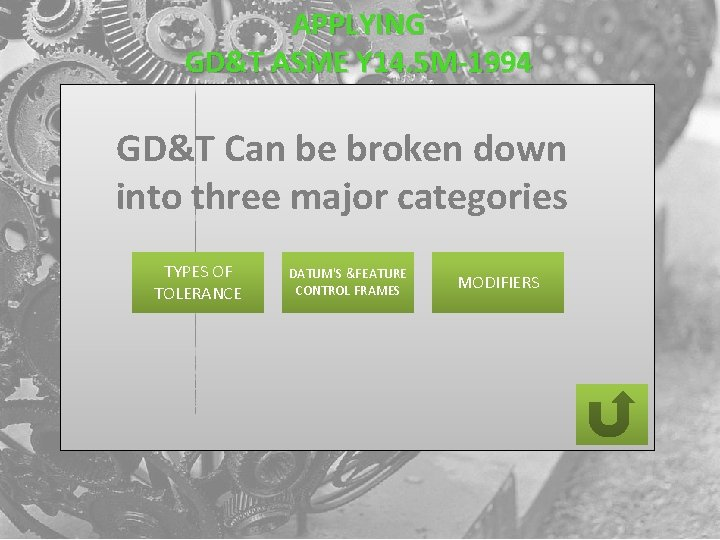APPLYING GD&T ASME Y 14. 5 M-1994 GD&T Can be broken down into three major categories TYPES OF TOLERANCE DATUM'S &FEATURE CONTROL FRAMES MODIFIERSTYPES OF TOLERANCE FORM PROFILE ORIENTATION Geometric tolerances that limit the amount of error in the shape of a feature. Form tolerances are independent tolerances. Powerful geometric tolerances that control the size, location, orientation, and form of a feature. Profile tolerances can be either independent or related. Geometric tolerances that limit the direction, or orientation, of a feature in relation to other features. Orientation tolerances are related tolerances. LOCATION Geometric tolerances that limit the location or placement of features. Location tolerances are related tolerances. RUNOUT Geometric tolerances that simultaneously limit the form, location, and orientation of cylindrical parts. Runout tolerances are related tolerances requiring a datum axis.FORM TOLERANCE STRAIGHTNESS A two-dimensional geometric tolerance that controls how much a feature can deviate from a straight line. FLATNESS A three-dimensional geometric tolerance that controls how much a feature can deviate from a flat plane. CIRCULARITY A two-dimensional geometric tolerance that controls how much a feature can deviate from a perfect circle. CYLINDICITY A three-dimensional geometric tolerance that controls how much a feature can deviate from a perfect cylinder.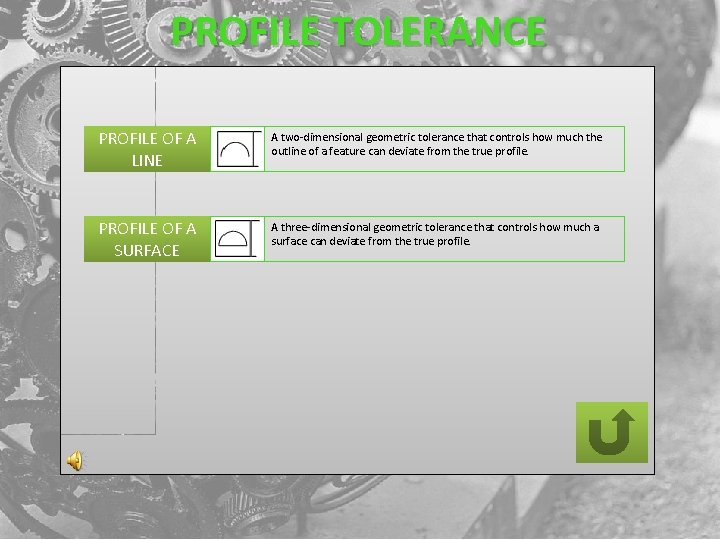PROFILE TOLERANCE PROFILE OF A LINE A two-dimensional geometric tolerance that controls how much the outline of a feature can deviate from the true profile. PROFILE OF A SURFACE A three-dimensional geometric tolerance that controls how much a surface can deviate from the true profile.ORIENTATION TOLERANCE ANGULARITY Perpendicularity Parallelism A three-dimensional geometric tolerance that controls how much a surface, axis, or plane can deviate from the angle described in the design specifications. A three-dimensional geometric tolerance that controls how much a surface, axis, or plane can deviate from a 90 degree angle. A three-dimensional geometric tolerance that controls how much a surface, axis, or plane can deviate from an orientation parallel to the specified datum.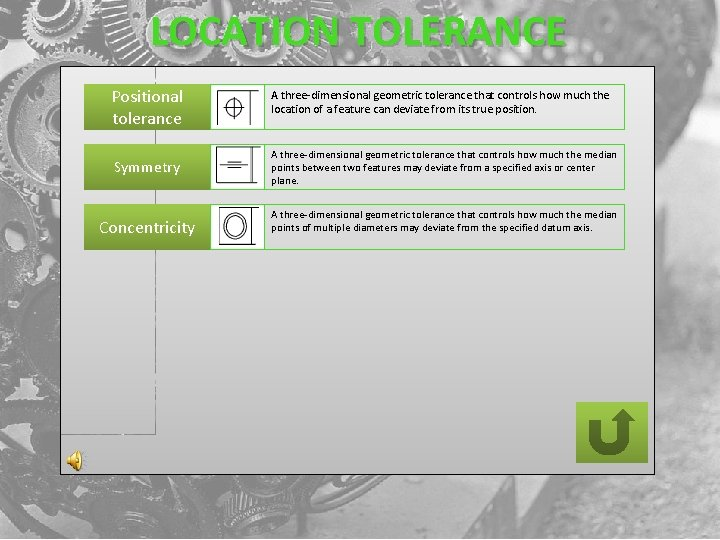LOCATION TOLERANCE Positional tolerance A three-dimensional geometric tolerance that controls how much the location of a feature can deviate from its true position. Symmetry A three-dimensional geometric tolerance that controls how much the median points between two features may deviate from a specified axis or center plane. Concentricity A three-dimensional geometric tolerance that controls how much the median points of multiple diameters may deviate from the specified datum axis.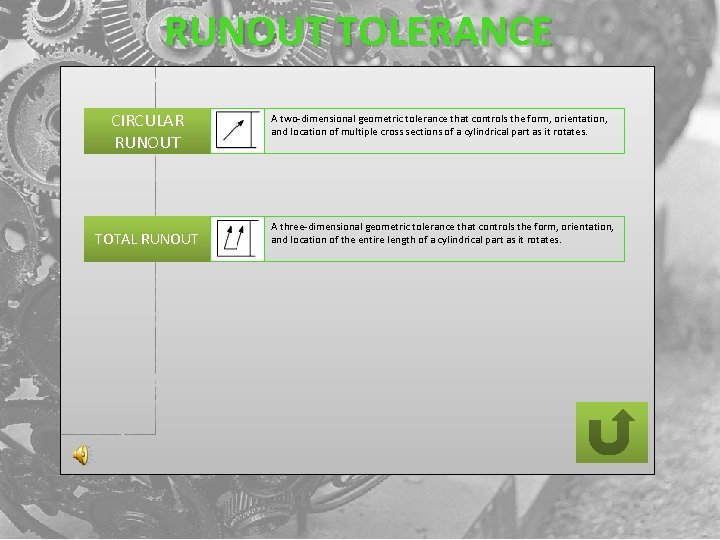RUNOUT TOLERANCE CIRCULAR RUNOUT TOTAL RUNOUT A two-dimensional geometric tolerance that controls the form, orientation, and location of multiple cross sections of a cylindrical part as it rotates. A three-dimensional geometric tolerance that controls the form, orientation, and location of the entire length of a cylindrical part as it rotates.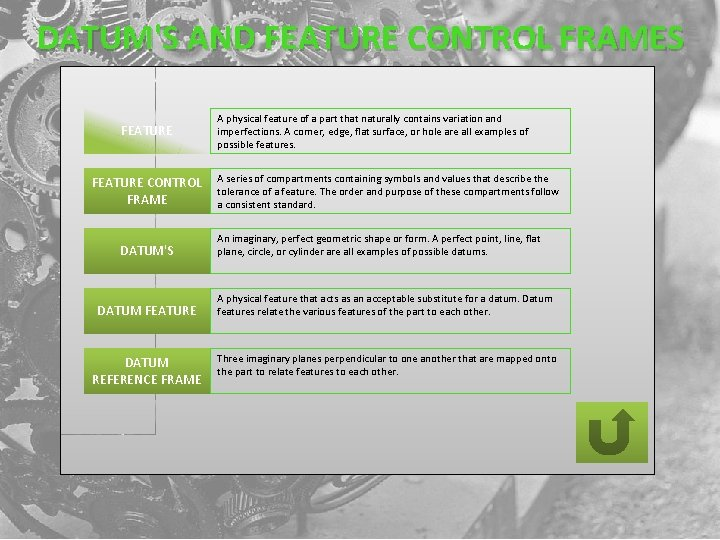DATUM'S AND FEATURE CONTROL FRAMES FEATURE CONTROL FRAME DATUM'S DATUM FEATURE DATUM REFERENCE FRAME A physical feature of a part that naturally contains variation and imperfections. A corner, edge, flat surface, or hole are all examples of possible features. A series of compartments containing symbols and values that describe the tolerance of a feature. The order and purpose of these compartments follow a consistent standard. An imaginary, perfect geometric shape or form. A perfect point, line, flat plane, circle, or cylinder are all examples of possible datums. A physical feature that acts as an acceptable substitute for a datum. Datum features relate the various features of the part to each other. Three imaginary planes perpendicular to one another that are mapped onto the part to relate features to each other.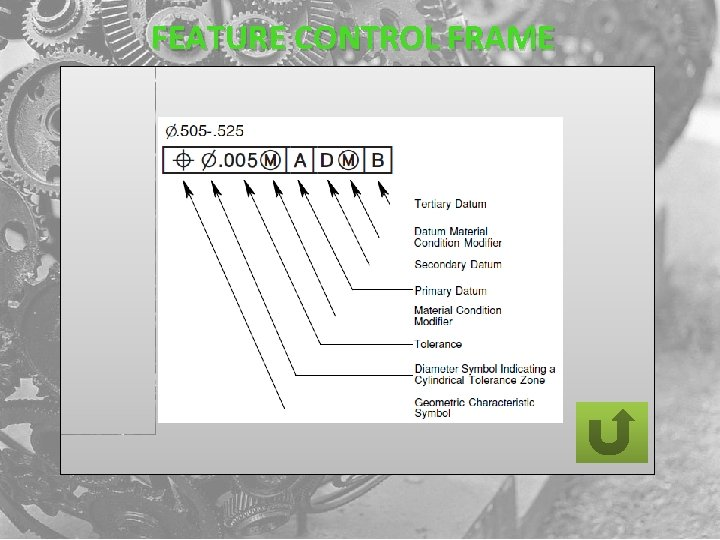FEATURE CONTROL FRAMEDATUM'S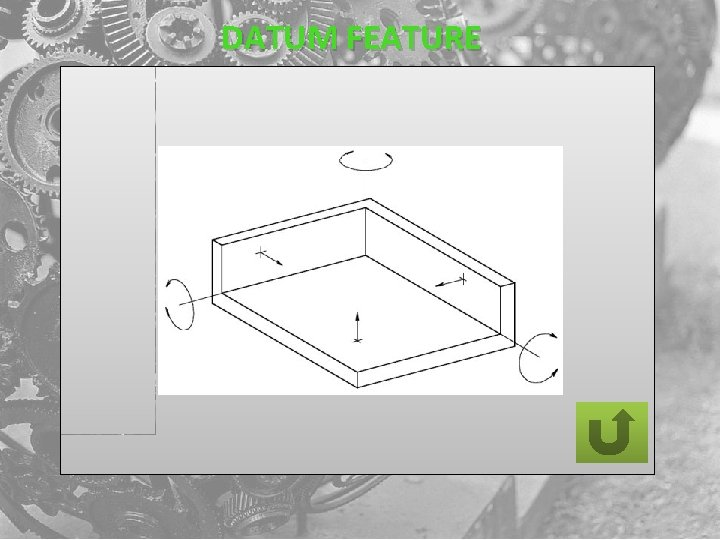DATUM FEATURE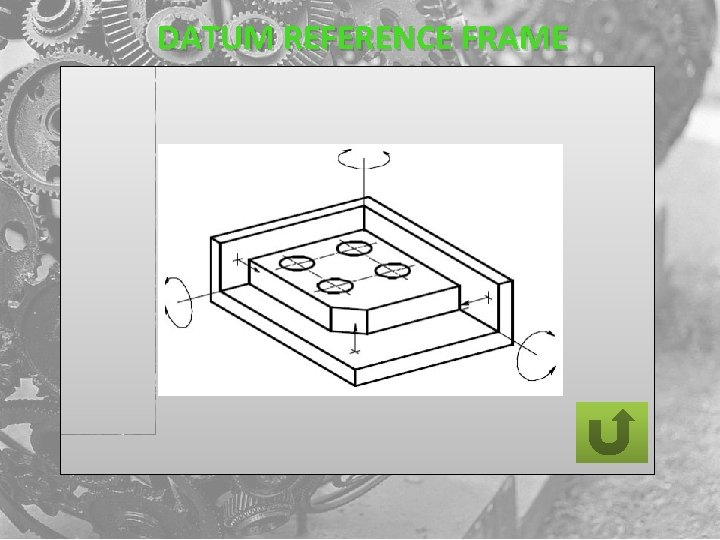DATUM REFERENCE FRAME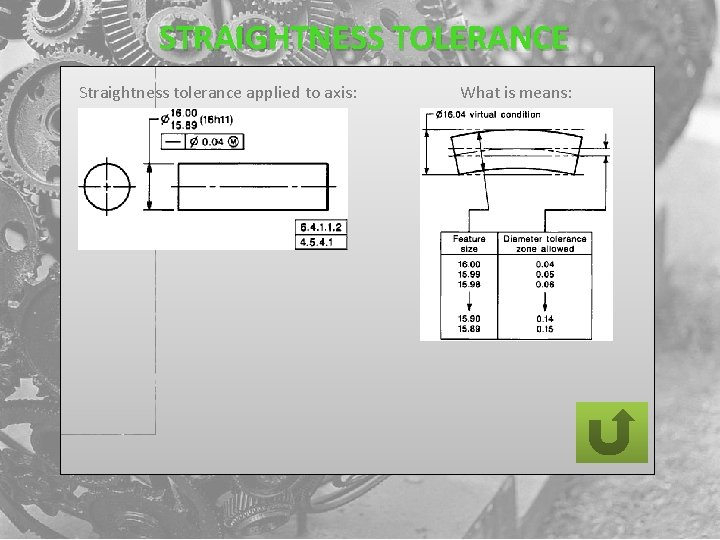STRAIGHTNESS TOLERANCE Straightness tolerance applied to axis: What is means: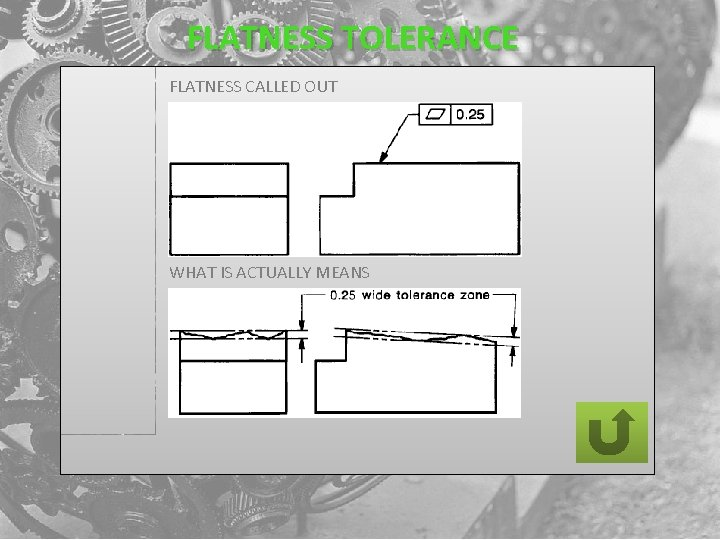FLATNESS TOLERANCE FLATNESS CALLED OUT WHAT IS ACTUALLY MEANS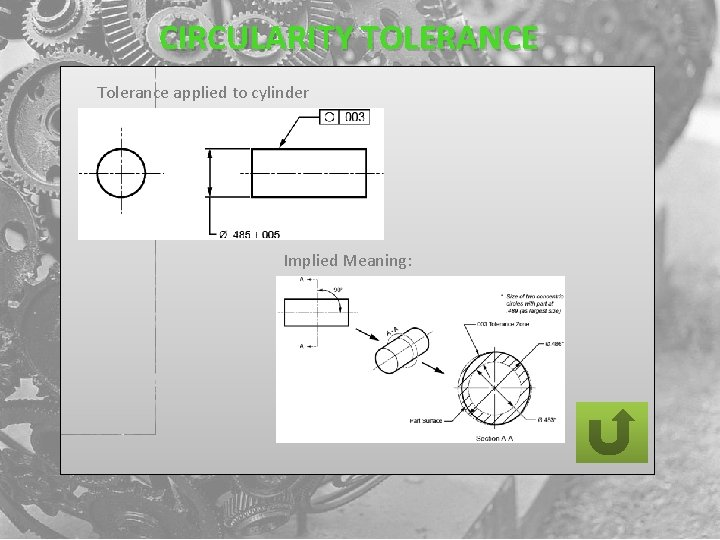CIRCULARITY TOLERANCE Tolerance applied to cylinder Implied Meaning: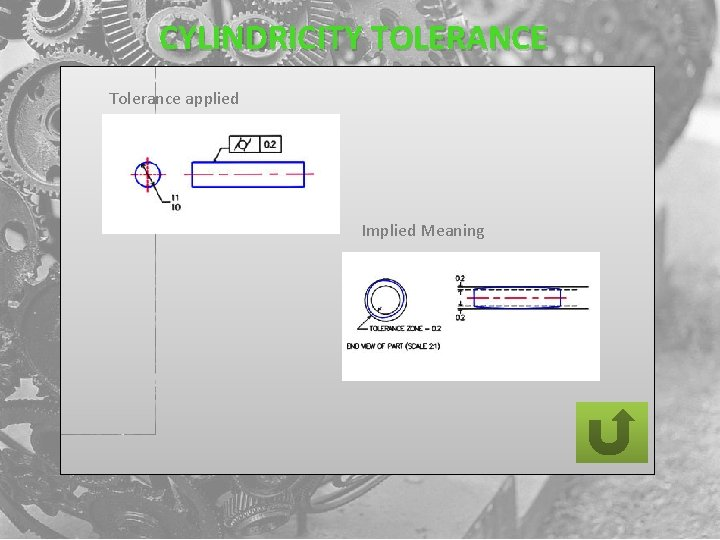CYLINDRICITY TOLERANCE Tolerance applied Implied Meaning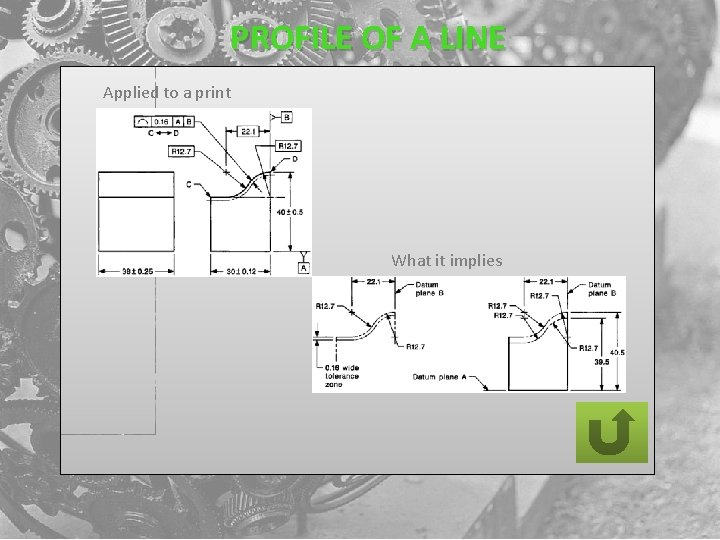PROFILE OF A LINE Applied to a print What it implies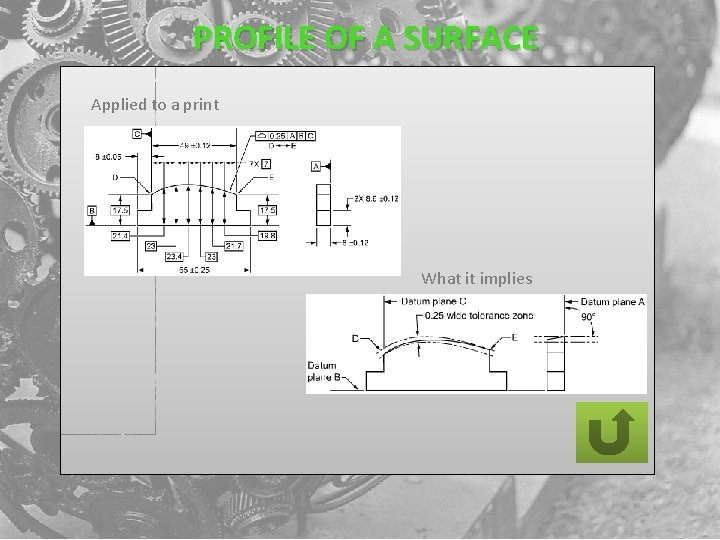PROFILE OF A SURFACE Applied to a print What it impliesANGULARITY TOLERANCE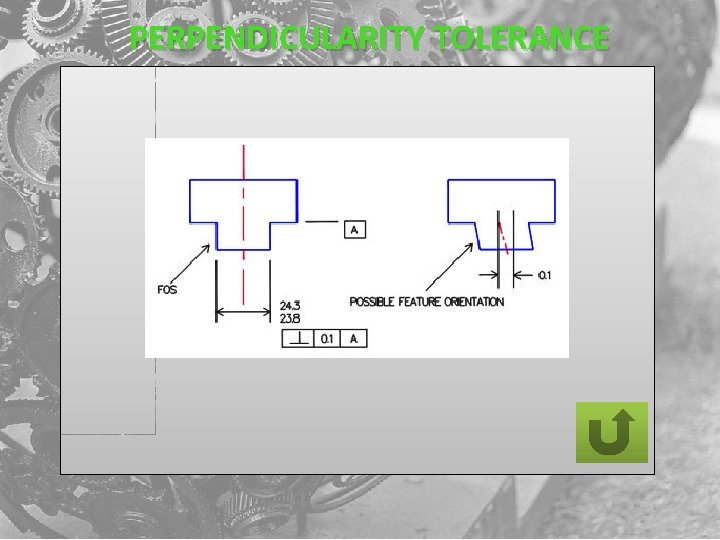PERPENDICULARITY TOLERANCEPARALLELISM TOLERANCE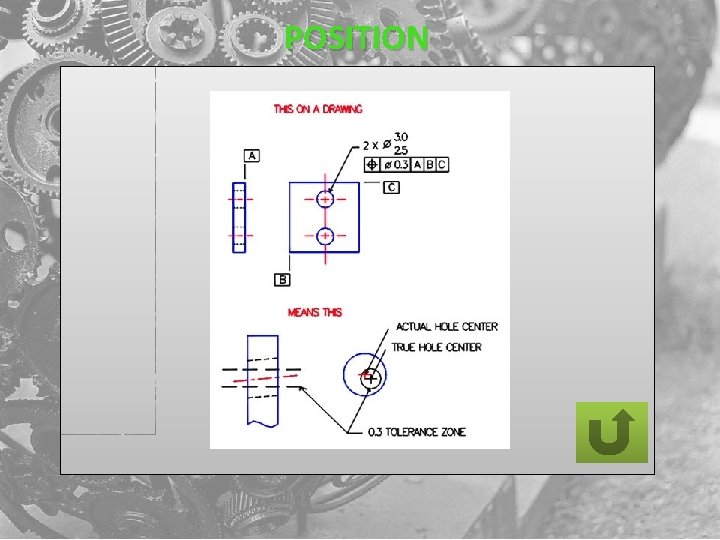POSITION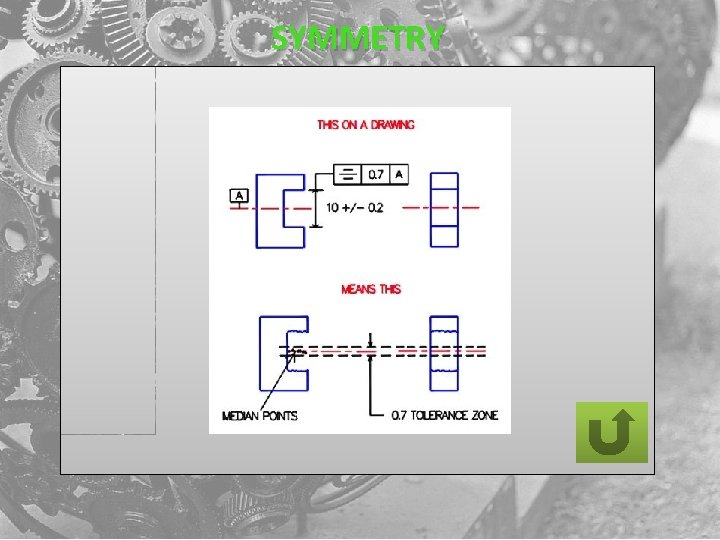SYMMETRY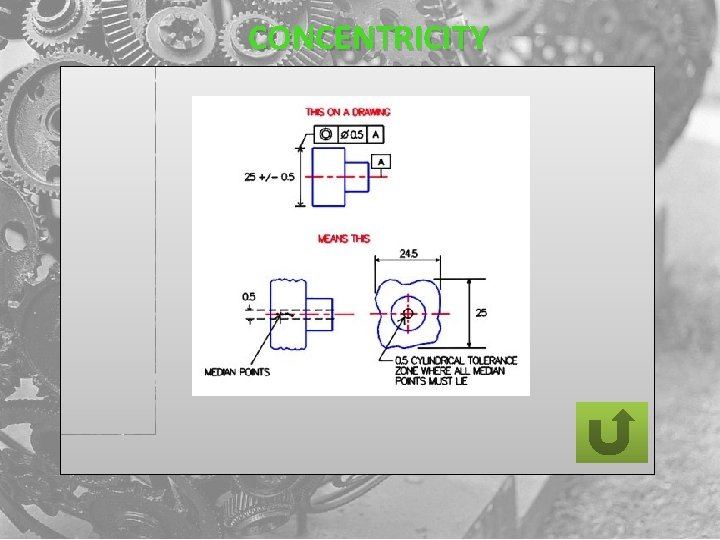CONCENTRICITY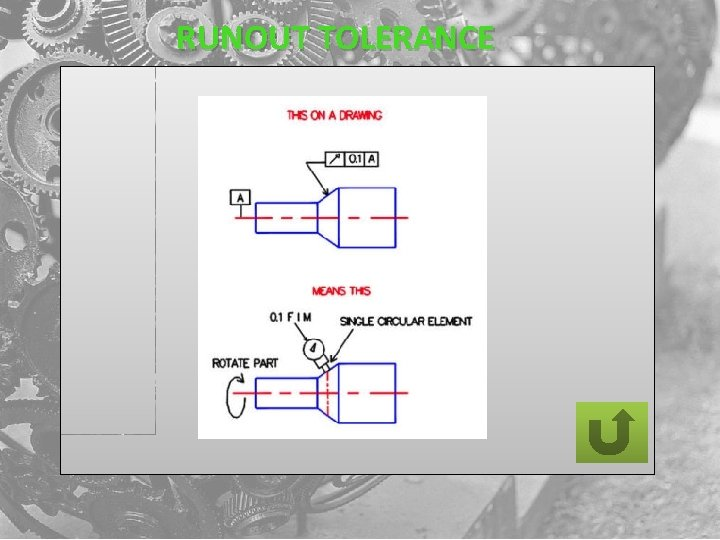RUNOUT TOLERANCE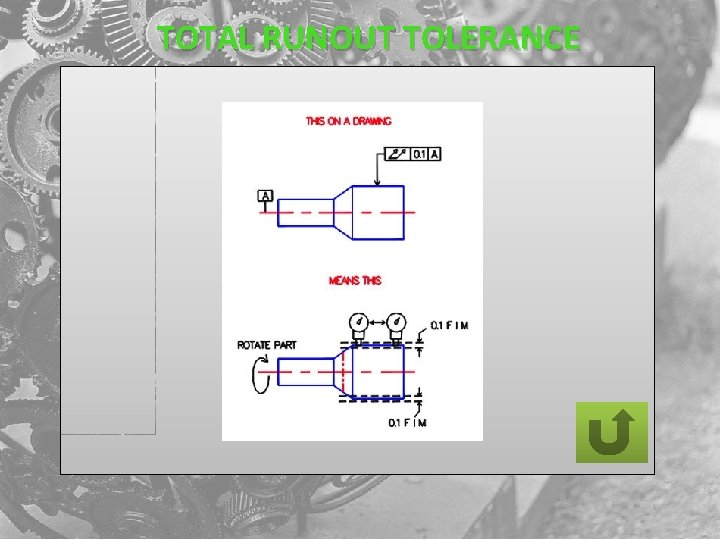TOTAL RUNOUT TOLERANCE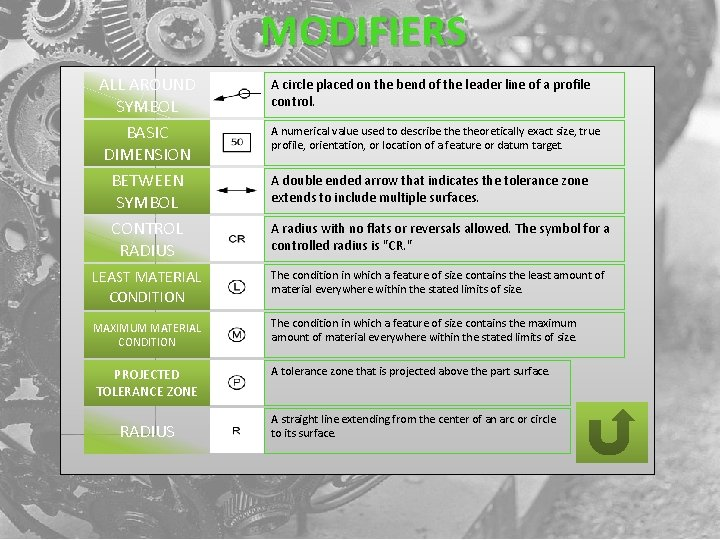MODIFIERS ALL AROUND SYMBOL BASIC DIMENSION BETWEEN SYMBOL CONTROL RADIUS A circle placed on the bend of the leader line of a profile control. A numerical value used to describe theoretically exact size, true profile, orientation, or location of a feature or datum target. A double ended arrow that indicates the tolerance zone extends to include multiple surfaces. A radius with no flats or reversals allowed. The symbol for a controlled radius is "CR. " LEAST MATERIAL CONDITION The condition in which a feature of size contains the least amount of material everywhere within the stated limits of size. MAXIMUM MATERIAL CONDITION The condition in which a feature of size contains the maximum amount of material everywhere within the stated limits of size. PROJECTED TOLERANCE ZONE A tolerance zone that is projected above the part surface. RADIUS A straight line extending from the center of an arc or circle to its surface.BASIC DIMENSION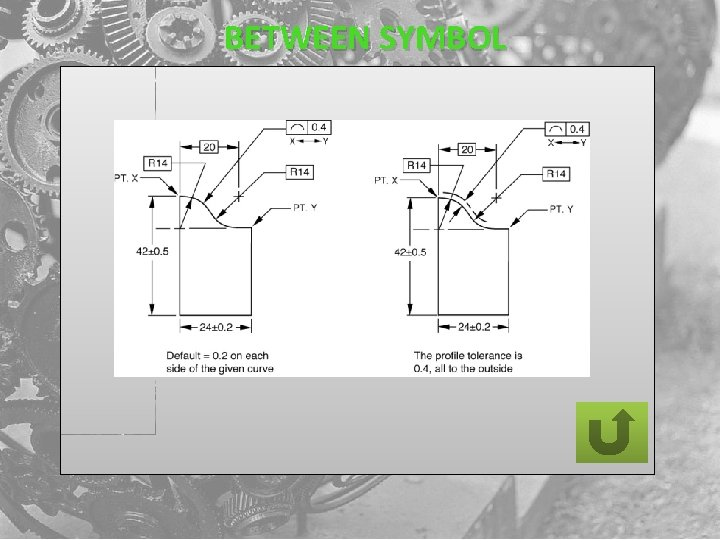BETWEEN SYMBOL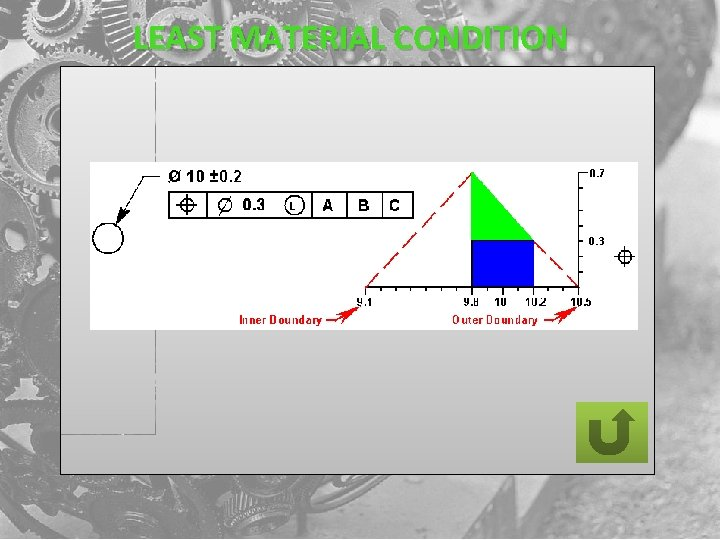LEAST MATERIAL CONDITIONMAXIMUM MATERIAL CONDITION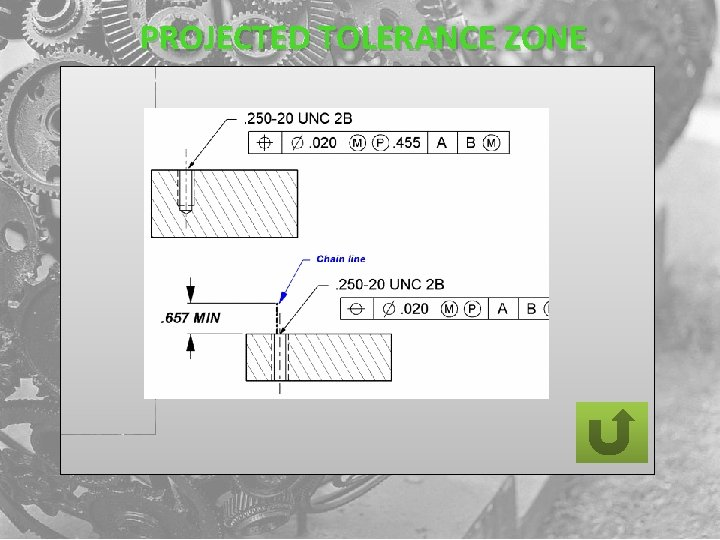PROJECTED TOLERANCE ZONEQUESTION 1 CONCENTRICITY IS A FORM TOLERANCE Concentricity TRUE FALSE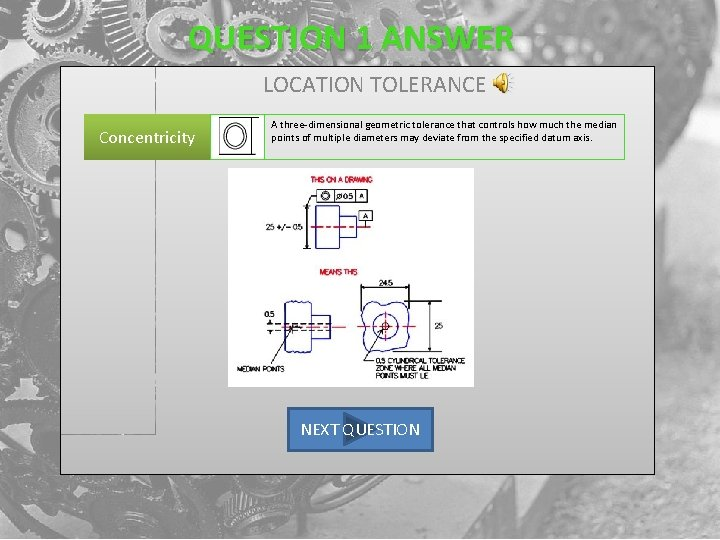QUESTION 1 ANSWER LOCATION TOLERANCE Concentricity A three-dimensional geometric tolerance that controls how much the median points of multiple diameters may deviate from the specified datum axis. NEXT QUESTIONOOPS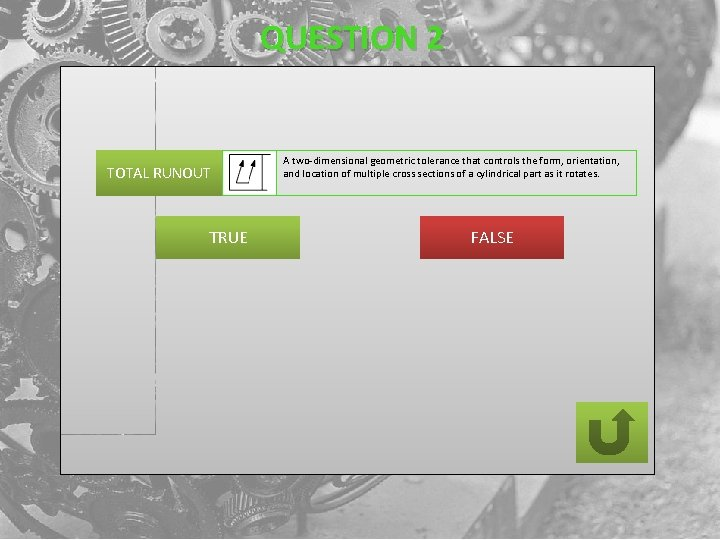QUESTION 2 TOTAL RUNOUT TRUE A two-dimensional geometric tolerance that controls the form, orientation, and location of multiple cross sections of a cylindrical part as it rotates. FALSEQUESTION 2 ANSWER GOOD WORK TOTAL RUNOUT A three-dimensional geometric tolerance that controls the form, orientation, and location of the entire length of a cylindrical part as it rotates. NEXT QUESTION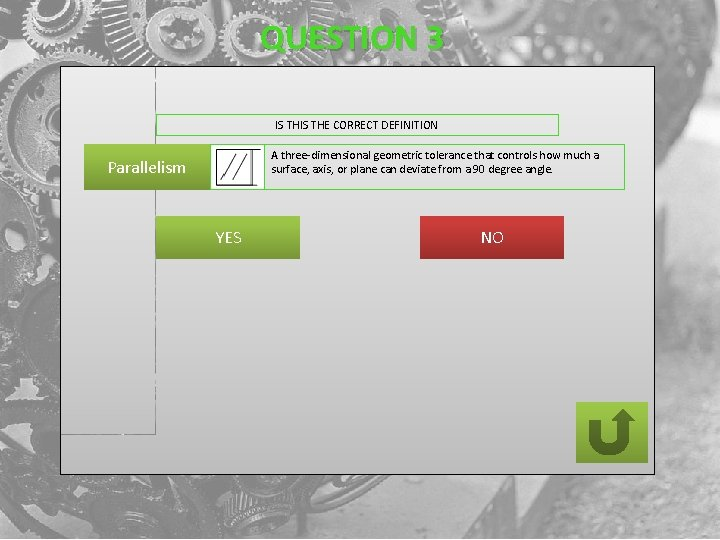QUESTION 3 IS THE CORRECT DEFINITION A three-dimensional geometric tolerance that controls how much a surface, axis, or plane can deviate from a 90 degree angle. Parallelism YES NOQUESTION 3 ANSWER GOOD WORK Parallelism A three-dimensional geometric tolerance that controls how much a surface, axis, or plane can deviate from an orientation parallel to the specified datum. NEXT QUESTIONQUESTION 4 GD&T PROVIDES A DESIGNER AN IMPROVEMENT IN THE USEABLE TOLERANCE ZONE OF 52% TRUE FALSE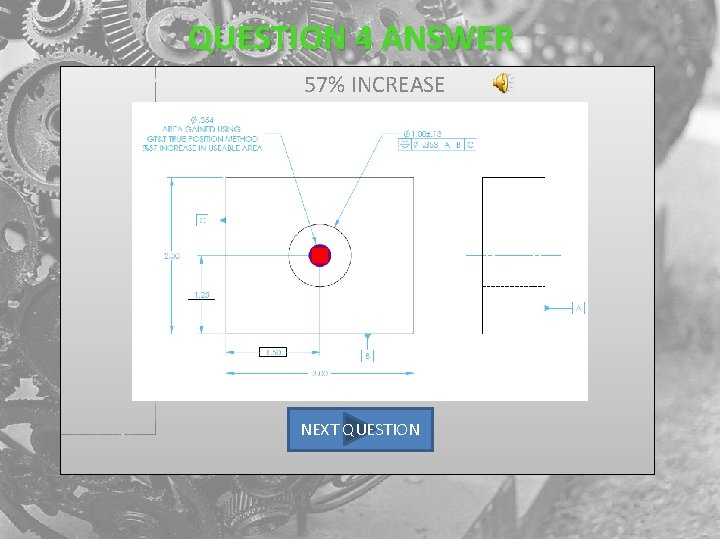QUESTION 4 ANSWER 57% INCREASE NEXT QUESTION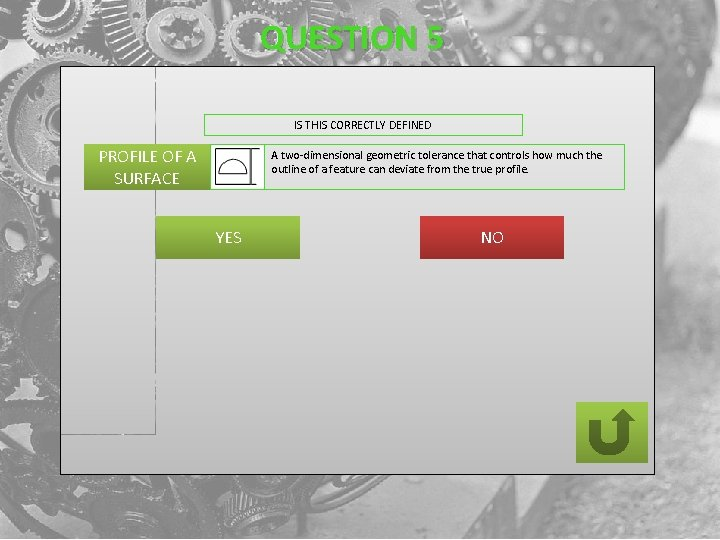QUESTION 5 IS THIS CORRECTLY DEFINED PROFILE OF A SURFACE A two-dimensional geometric tolerance that controls how much the outline of a feature can deviate from the true profile. YES NO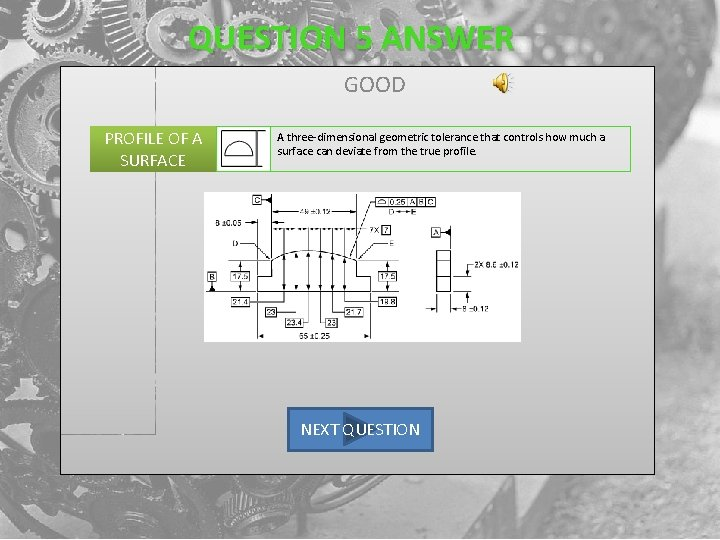QUESTION 5 ANSWER GOOD PROFILE OF A SURFACE A three-dimensional geometric tolerance that controls how much a surface can deviate from the true profile. NEXT QUESTIONQUESTION 6 TRUE FALSE THIS IS AN EXAMPLE OF A DATUM REFERENCE FRAME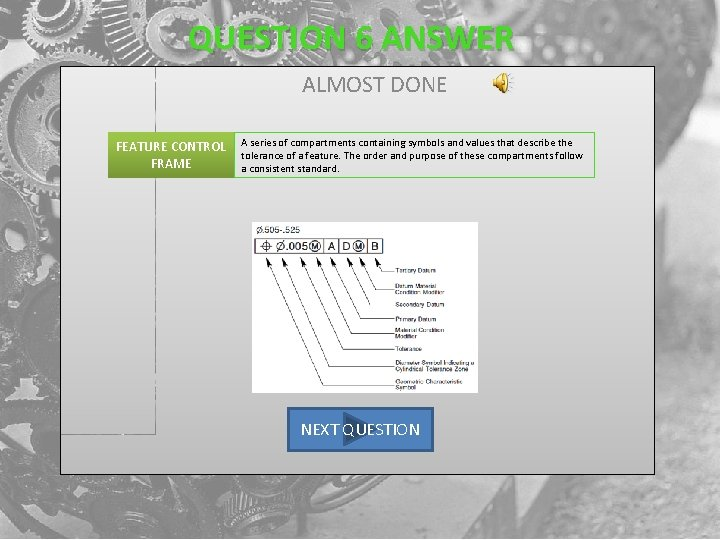QUESTION 6 ANSWER ALMOST DONE FEATURE CONTROL FRAME A series of compartments containing symbols and values that describe the tolerance of a feature. The order and purpose of these compartments follow a consistent standard. NEXT QUESTIONQUESTION 7 IS THE CORRECT SYMBOL FOR CIRCULARITY TRUE FALSE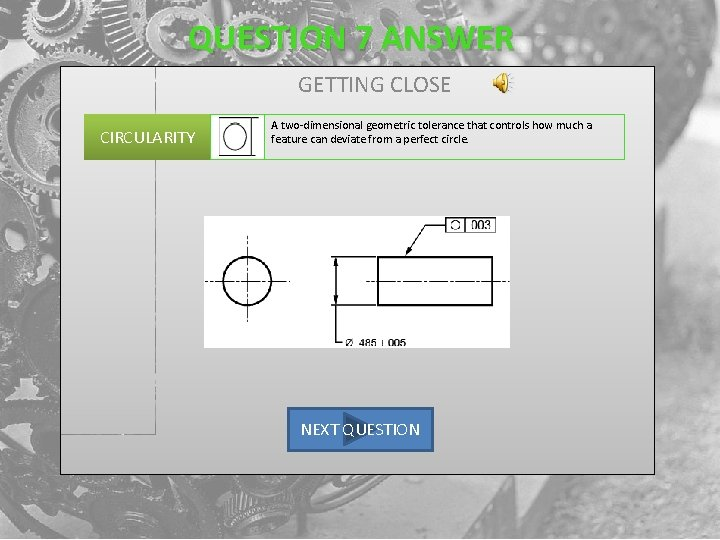QUESTION 7 ANSWER GETTING CLOSE CIRCULARITY A two-dimensional geometric tolerance that controls how much a feature can deviate from a perfect circle. NEXT QUESTIONQUESTION 8 IS THIS CORRECTLY DEFINED DATUM'S An imaginary, perfect geometric shape or form. A perfect point, line, flat plane, circle, or cylinder are all examples of possible datums. YES NO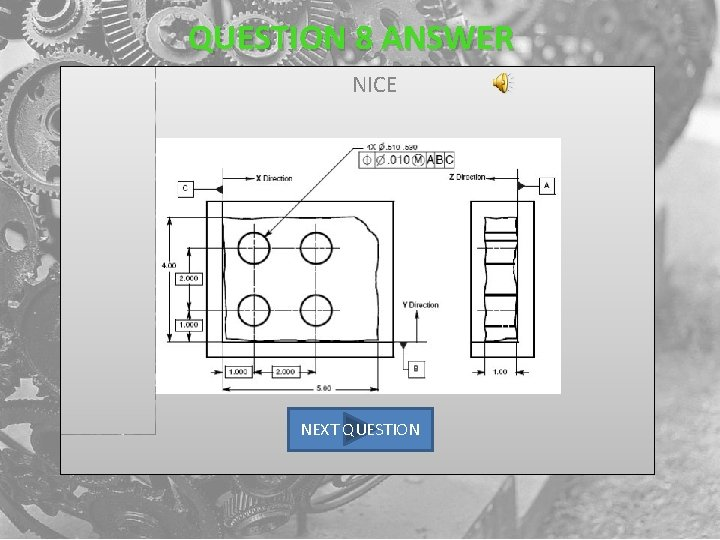QUESTION 8 ANSWER NICE NEXT QUESTIONQUESTION 9 IS THE CORRECT SYMBOL FOR STRAIGHTNESS A two-dimensional geometric tolerance that controls how much a feature can deviate from a straight line. STRAIGHTNESS YES NO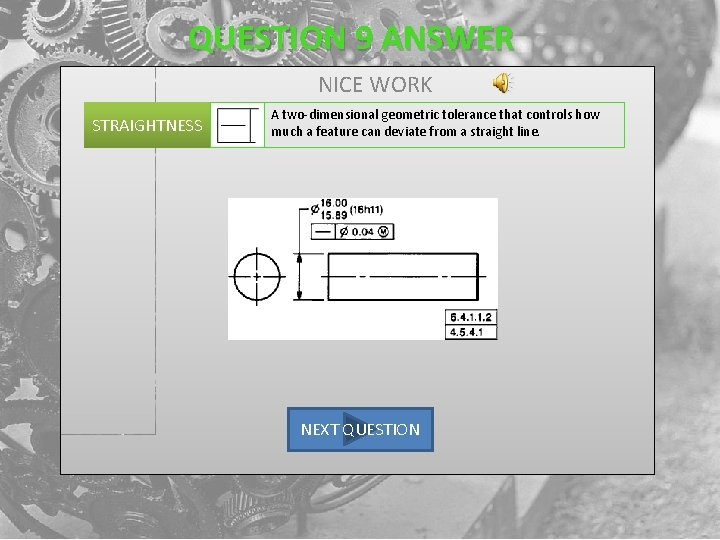QUESTION 9 ANSWER NICE WORK STRAIGHTNESS A two-dimensional geometric tolerance that controls how much a feature can deviate from a straight line. NEXT QUESTION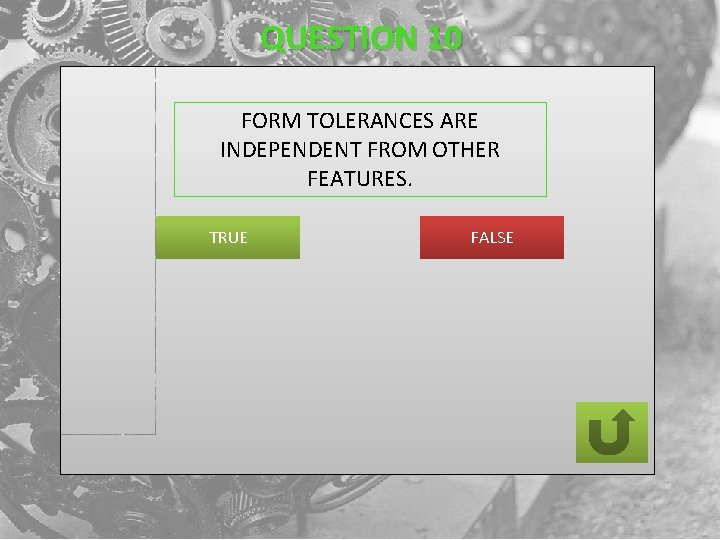QUESTION 10 FORM TOLERANCES ARE INDEPENDENT FROM OTHER FEATURES. TRUE FALSEQUESTION 10 ANSWER CONGRATULATIONS PLEASE SEE THE INSTRUCTOR FOR YOU FIRST ASSIGNMENT FINISH